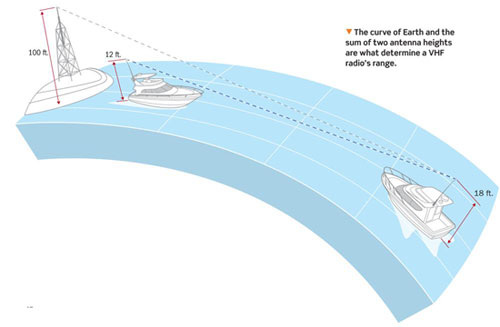### How far can a VHF radio reach?

2021-03-17

RADAR HORIZONHow far can a VHF radio reach?

The range of a radio is based on multiple factors such as the power of your radio and even atmospheric conditions, but in an ideal situation we can determine the maximum range of a VHF radio using a simple equation!

The Radar Horizon is essentially the line of sight from the radar (or VHF radio) to the horizon. The transmission does not penetrate the earth, so the distance a radio can reach is limited by the curvature of the earth.

The distance to the horizon is calculated by multiplying the square root of the antenna's height in feet by 1.23 with the result in nautical miles.

(√antenna height) * 1.23 = radar horizon

In the example taken from the image above we see the ship on the right has an antenna 18ft high so:

( √18ft) * 1.23 = 5.22nm

Therefore, with enough power the antenna can transmit to a station 5.22nm away

But what if we want to make a ship-to-ship transmission?

We then need to take into account the height of both antennas, so we modify the equation like so:

The maximum range to a target is calculated by adding the square root of the height of one antena to the square root of the  height of the target and then multiplying the result by 1.23. Don't forget about BEDMAS!

(√18 + √12) * 1.23 = 9.48nm

Now it's your turn!

Determine the maximum range that each ship will have when communicating with the 100ft tall shore station antenna!

Source of the materials：